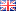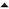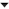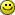## Fenwick trees demystifiedA Fenwick tree is a clever way to represent a list of numbers in an array, which allows arbitrary-length prefix sums to be calculated efficiently. (For example, the list [1,2,3,4,5] has a length-3 prefix [1,2,3] with sum 1+2+3 = 6.) This is useful in various scenarios, most notably to implement arithmetic coding, which requires dynamic tracking of cumulative frequencies of previously encountered symbols.

The data structure was introduced by Peter Fenwick in 1994, in a report titled “A new data structure for cumulative frequency tables”. Fenwick called it a Binary Indexed Tree, after the observation that the binary representation of indices determines the implicit tree structure, but the term Fenwick tree seems to be more popular today. Many articles are already available online that explain how a Fenwick tree may be implemented. Unfortunately, these articles invariably fail to explain how it was derived.

Fenwick himself shares the responsibility for the confusion, since he did not bother to discuss the history of the data structure in his publication. This has lead readers to believe there is something magical about the particular layout that is used, and caused programmers to blindly copy the source code from Fenwick's report or another source, instead of trying to understand the underlying principle first and then deriving the necessary code themselves. That's a pity, since proper understanding of a solution is necessary to extend, adapt or reconstruct it.

In this article I will try to fill this gap in public knowledge by explaining how the Fenwick tree structure and the algorithms that operate on it can be derived from scratch.

#### The problem

The problem under consideration is as follows. Suppose that we have a numeric array a, and we want to be able to perform two operations on it: either changing the value stored at an index i, or calculating the sum of a prefix of length n.

A straight-forward implementation would look like this:
JavaScript:
 ```1 2 3 4 5 6 7 8 9 10 11 12 13 14 ``` ```// An array with some initial values: a = [ 3, 1, 4, 1, 5, 9, 2, 6 ] // Update: add v to a[i] function update(i, v) { a[i] += v } // Prefix sum: calculate the sum of a[i] for 0 <= i < n. function prefixsum(n) { var sum = 0; for (var i = 0; i < n; ++i) sum += a[i] return sum }```

This is a perfectly reasonable solution, but unfortunately the time required to calculate a prefix sum is proportional to the length of the prefix, so this may be rather slow in practice.

A Fenwick tree allows prefix sums to be calculated much faster (while making updates a little slower). Formally, each operation can be performed in O(log n) time using a Fenwick tree, instead of O(1) and O(n) time for updates and queries respectively. The twist: unlike most other data structures that solve this problem, Fenwick trees require no additional memory!

Of course, this is a surprising and exciting prospect. Before explaining how it works, I will first present a data structure that achieves the same complexity bounds, but uses twice as much memory as before. Then I will show how the compact representation that Fenwick trees are known for can be derived by combining all data into a single array.

#### An intermediate representation

Consider, as an example, an array a of length 8. We can speed up prefix sum calculations by grouping the elements of the array by pairs, and storing the sum of each pair in a new array of length 4. Then we can repeat the procedure on that new array to obtain an array of length 2, and so on. This is illustrated in the figure below:

The result is a two-dimensional array-of-arrays called A (where A contains the original array a). We can use this to calculate prefix sums quickly, by taking at most one element from each row, and adding these values together. For example, the sum of the first five elements can be calculated as: A + A == 5 + 9 == 14.

It is easy to tell visually which blocks must be added together to find a prefix sum. The process can be described more formally as follows. Start at row r=0 with prefix length n. If n is odd, then we need to add A[r]\[n-1] to the sum. In any case, we divide n by 2 and move down a row by incrementing r to 1. Then, we check again if n is odd, and if so, we add A[r]\[n-1]. This process is repeated until the bottom of the table is reached.

Updating values works in a similar manner: if we add/subtract a value in the original array, we must add/subtract that value to all blocks in the same column (one block per row) to keep the calculated sums consistent. Since every block is twice as wide as the one before it, we need to divide the index by two for each row.

These operations may be implemented as follows:
JavaScript:
 ```1 2 3 4 5 6 7 8 9 10 11 12 13 14 15 ``` ```function update(i, v) { for (var r = 0; r < A.length; ++r) { A[r][i] += v; i >>= 1; } } function prefixsum(n) { var sum = 0; for (var r = 0; r < A.length; ++r) { if (n&1) sum += A[r][n - 1]; n >>= 1; } return sum; }```

(Note that >> is the right-shift operator, which is used here to perform integer division while rounding down.)

This intermediate representation already provides the O(log n) time bounds that we desired, which follows from the fact that we need only 2log n + 1 rows for an array of n elements and the observation that we access (at most) one element in each row for each operation. The only problem left is that this representation requires twice as much memory to store the array-of-arrays A.

#### Optimizing for space

So where does Fenwick's space optimization come from? If we look carefully at the code used to calculate prefix sums, we see that we only access array elements at even indices, since we first check if n is odd (n&1) and if so, we add the element at index n-1 of the corresponding row, which must therefore be even.

That means that for each row we store, half the elements in the array are completely useless: the elements at odd indices are never used to calculate prefix sums! We can recycle this wasted space by storing the elements of A\ at the odd indices of A\, storing the elements of A\ at the odd indices of A\, and so on, until all relevant data is actually stored in the topmost array.

This compact array representation is called a Fenwick tree (though arguably Fenwick array would have been a more appropriate term) and is illustrated in the following figure:

This representation is nice because it allowed us to reduce the array-of-arrays A to a single, flat array a, no larger than the original array, yet we can still calculate prefix sums by adding the appropriate values, though they are now stored at different locations:
JavaScript:
 ```1 2 3 4 5 6 7 8 9 ``` ```prefixsum(0) == 0 prefixsum(1) == a == a + prefixsum(0) prefixsum(2) == a == a + prefixsum(0) prefixsum(3) == a + a == a + prefixsum(2) prefixsum(4) == a == a + prefixsum(0) prefixsum(5) == a + a == a + prefixsum(4) prefixsum(6) == a + a == a + prefixsum(4) prefixsum(7) == a + a + a == a + prefixsum(6) prefixsum(8) == a == a + prefixsum(0)```

In general: the prefix sum of length n > 0 can be calculated as a[n - 1] + prefixsum(n&(n-1)), where & is the bitwise AND-operator which is used here to clear the least-significant bit in the binary representation of n. The translation to source code is straightforward:
JavaScript:
 ```1 2 3 4 5 6 7 8 9 ``` ```function update(i, v) { while (i < a.length) a[i] += v, i |= i + 1; } function prefixsum(n) { var sum = 0; while (n > 0) sum += a[n - 1], n &= n - 1; return sum; }```

Note that this implementation is both simple and highly symmetric. In the implementation of update, 1-bits are added to the index i until it exceeds the size of the array, while in the implementation of prefixsum, 1-bits are stripped from n until it becomes 0. Beautiful, isn't it?

#### Conclusion

At this point, it should be clear not only how Fenwick trees can be used to efficiently calculate prefix sums in dynamic arrays, but also how their structure can be derived from a more obvious intermediate representation; a crucial piece of information that is missing from other articles about Fenwick trees. Hopefully, this will demystify the origin of this data structure, and lead to both deeper understanding and greater appreciation of Fenwick's admittedly clever invention.

Two notes on the implementation presented here:
1. In the original report, Fenwick treats the value stored at index 0 as an exception. This unnecessarily complicates the implementation and is obviously ugly. I have fixed this shortcoming in this article.
2. Although I have used an example array with size 8 which is conveniently a power of 2, it should be noted that the source code presented here works correctly on arrays of any size.
Finally, I have only covered the two essential operations on Fenwick trees (update and prefixsum). Many more are possible and sometimes required. Readers who enjoy a hand-on approach to learning about data structures are encouraged to try and solve the following problems themselves:
1. How can we retrieve the value stored at a particular position? For even indices, the answer is simple, but for odd indices, more effort is required to reconstruct the original value. (Fenwick's report also contains an answer to this question.)
2. Given a Fenwick array, how can we extend it by adding values at the end?
3. How can we implement the inverse of the prefixsum() function? That is, given a sum s, how can we find the smallest prefix length n such that prefixsum(n) >= s? (This functionality is used in the implementation of arithmetic decoders.)01-'15 Facebook Hacker Cup 2015: Qualification Round problem analysis03-'13 Cyber Crime Challenge 0xFFD

A very clear explanation for a phenomenon I didn't know aboutThe two colored graphs combined clearly represent how you can eliminate the data that can be derived and squash the two-dimensional array down into one.

As a professional software developer (business applications) I just don't see any real world example where one would need such an algorithm. Maybe you could elaborate on that?

The main practical application of Fenwick trees I know of, is to speed up dynamic arithmetic coding/decoding (also range coding, which is basically the same), since this requires tracking symbol frequencies dynamically.

The topic is moderately specialistic, and mainly of interest to three kinds of programmers:
• Library implementers.
• Computer scientists.
• Those who find algorithmic problems intrinsically interesting.
Business software development focusses more on business logic and less on implementation details. Sophisticated data structures are used only through libraries that hide their implementation from their users. That's a perfectly reasonable and pragmatic approach, and it means you will probably never find the need to implement a B-tree or sorting algorithm from scratch yourself. In that case, the kind of weblog posts I write may be of little interest to you.

However, if you are interested in Fenwick trees at all (for whatever reason), then I think it is important that you try to understand where they come from as well as how they work. Previous articles explained how but not so much why they work. I wrote this article to explain what the crucial insight behind Fenwick trees was.

If you start out with an input array of n elements, what is the cost of constructing the Fenwick tree? Is it O(n) or O(n log n)? The latter is possible by simply initializing it to 0, and updating all elements. But perhaps there is a more efficient way?

The Fenwick tree can be constructed from an arbitrary array in linear time, by observing that the intermediate representation adds less than n elements in total (since the limit of n/2 + n/4 + n/8 ... etc. is equal to n), and each element can be calculated with a single addition.

This can be translated to the compact representation too, by iterating over rows and performing the necessary additions row-by-row. For example, as follows:

JavaScript:
 ```1 2 3 4 5 ``` ```for (var i = 1; i < a.length; i = 2*i) { for (var j = 2*i - 1; j < a.length; j += 2*i) { a[j] += a[j - i] } }```

edit:
Similarly, the Fenwick tree can be converted back to the original array in O(n) by running the same algorithm from bottom to top.

[Comment edited on zondag 26 januari 2014 18:25]